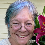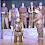## 29 April 2012

### Math puzzle

Three spaceships land randomly on a spherical planet.

What is the probability that all three will land in the same hemisphere?

100%.  As explained at Futility Closet, "Point A, Point B, and the planet’s center determine a plane that cuts the planet in half; Point C must lie on one side or the other of this plane (or directly on the great circle)."

(I suppose someone can argue whether three points on a great circle are in the same hemisphere...)

Image credit.

1.Good grief, that's so trivial I assumed that the question was about pre-defined hemispheres, viz. northern or southern, etc.

Plus, from an aeronautical point of view isn't it far more difficult to make planetfall unless you do so on the equator? At any other angle you are going to face a higher risk of bouncing off the atmosphere or losing a substantial percentage of the angular momentum?

1.It may be a bit easier to calculate the trajectory of a spacecraft reentering above the equator (i.e. with 0° of inclination), since the Coriolis accelerations (due to the rotation of the planet) wouldn't have to be considered.
I don't think reentry is any more difficult or risky at higher inclination angles though, because the speed of rotation of the atmosphere is negligible compared to the speed of the spacecraft.

2.Whatever, dude.

2.I thought the same thing as anon up there, that we were talking about southern/northern hemispheres. In which case I suppose the probability would be 1/8, right? (same as getting 3 heads in a row in a coin toss).
But yeah, if the hemispheres are defined by the first two spaceships, the problem becomes pretty trivial.

By the way, even if it can be argued that three points on a great circle don't belong to the same hemisphere, the result is still the same: the probability that the three spaceships land exactly on a great circle is 0.

3.Maybe I'm stupid but... if point A and point B on the surface are close together, how can the center of the planet have anything to do with them?

1.The closeness doesn't matter. Any two points on the surface of the earth (your two feet when standing up, or Louisville and Moscow), plus the center of the earth, will define a plane. Slice along that plane, and you cut the globe into two equal parts.

4.In reply to DHill - it would actually be 1/4 if you're talking about predefined hemispheres. The first spacecraft lands wherever, and that defines the hemisphere that the other two spacecraft have to land in as well. So it's 1/2 that the second spacecraft lands in the same hemisphere, and 1/2 that the third spacecraft also lands in the same hemisphere. It's like the question "What are the odds of tossing a coin three times and getting the same result (heads OR tails) each time".

1.Wrong. One spacecraft does not define a hemisphere. And two will always be on the same hemisphere.

2.Annon already said he was talking about "predefined" hemispheres (i.e. North and South)

So the spacecraft didn't define the hemisphere itself, but only which one the other ships were required to land in.

Dhill pointed out that the odds of getting 3 heads in a row are 1/8. BUT the odds of getting three coin flips in a row to be the same (all heads OR all tails) is 1/4. Annon simply pointed out the spaceship question was the second case, not the first.

3.You're right, I mistakenly calculated the odds of all spacecraft landing in one arbitrarily chosen hemisphere.
Thanks for that ;-)

5.I'm totally confused by this. If points A and B are on the line of an 'equator', then they are not really in one hemisphere or the other, unless they are in both. So the sphere cannot be divided into two hemispheres using this definition. The chances of the third point being in either hemisphere containing A or B are zero. Points A and B cannot be used to define the hemispheres at all. I thought a point has no dimensions and a line has only one dimension. So point A and B must be on one side of the line or the other but not on it. If they are on it then they are not in either hemisphere. Is that not so? An actual spacecraft would have volume and so touch both sides of the line and be in both hemispheres defined by their chosen touch down points.

1.Unless a spacecraft occupies zero physical space, it would be in both hemispheres at once. Even if there were such thing as a zero-point spacecraft, there isn't any no-man's-land area in the middle of the line.

6.I was also thinking north-south or east-west. So, if the first ship landed in the northern and western hemispheres, the second one would land in one of those hemisphere's 75% of the time (one time in 4 it would land in the southern and eastern hemispheres). The third ship would land in the same hemisphere as the other two half the time. So the probability all three would be in the same hemisphere (in this case, all north or all west) would be 3/8ths.

Having gotten that far, it should have occurred to me that hemisphere is arbitrary.

7.it is sweet

8.It's a "trick question" that plays on common definitions and perceptions:
"What is the probability that all three saucers will land in the same hemisphere as defined by the relative position of the saucers?" would be far more accurate but not a terribly interesting post.

9.it's like baseball...the
"foul line" is actually fair territory. If 2 ships land ON the line then they are within the boundaries of a hemisphere. and the third will inevitbly be within either half or on the line, in whitch case it is a "fair ball." btw, assuming predetermined hemispheres my math came to 3/8 but i could be wrong considering line is fair territory in either direction.